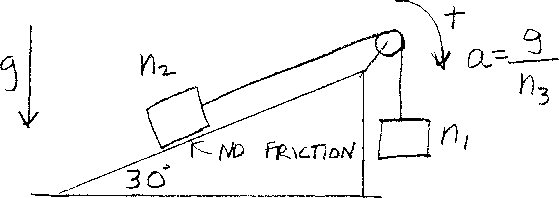Problem B6: Consider the two blocks shown in the figure above. A block of mass n2 can slide without friction on the incline. The incline makes an angle of 30 degrees with the horizontal. Another block of mass n1 is hanging from the cord. The cord goes over a massless pulley. Assume that the cord also is massless. Both blocks move with constant acceleration. The acceleration of the system is g/n3. What is n3? Note that positive acceleration is the direction shown by the arrow in the figure.n1 = n2 = Input n3:
If you are currently in my class, you can record your grade by entering your name and student ID number (without the leading zeros) below and clicking on "record grade".
 First Name = Last Name = ID = Problem: